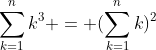## Monday, 17 January 2011

### Sum of Cubes, Square of sum..Potato/Po'ta toe?

Ok, here is an interesting trick; take any number and make a list of its factors (including one and itself). Now count the number of divisors each factor has (include one and the number itself) and put them in another list. Now cube each of the numbers in that new list, and also square the sum of the list. MAGIC???

Let me illustrate. If I pick 12 the factors are {1, 2, 3, 4, 6 and 12}. The number of factors of those numbers are {1, 2, 2, 3, 4, 6}. The magic part... 13+23+23+33+43+63=324... and (1+2+2+3+4+6)2= (18)2= 324...

This is related to the well known relation that for any string of consecutive integers {1,2,3, ...n} the sum of the cubes is equal to the square of the sum.(student's should prove this by induction).

I came across this recently at a blog called Alasdair's Musing. He gives credit to Joseph Liouville. He has a nice proof of the relation using Cartesian cross products of sets. Yes, children, you want to know what that means.